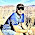How to

When solving these problems, learning by doing is much better than learning by reading. I encourage to you read only as far in the solution as you need, then trying to solve the problem. If you get stuck, try reading a little further. And of course, let me know if you find a better solution!

Tuesday, February 14, 2012

Remove Occurrences of a Character

Problem:  Given a null terminated c string char array, remove all occurrences of a specified char from it.

void removeOccurencesOfChar(char * a, char cToRemove){
...
}

Solution:
Let's start with a picture to make sure that we fully understand the problem.  Pic 1 is an example of this problem.

We have an array of chars which is null terminated in pic 1.  Let's assume that we we want to remove the character 'f' from this array.  At first, this seems like it is pretty straight forward.  We can just iterate through the array until we find  'f' , and then remove it from the array.

But, let's think about what it means to 'remove a character' from an array.  There are lots of ways that we could do this.  One obvious first way to do this is to shift the right side of the array after 'f', one character over.

If we had a linked list instead of an array, this would be trivial as we could fairly easily just re-arrange pointers.

But, this is an array, so we need to think a little bit more.  Well, we're already iterating through the array, so think about how a shift could be implemented in addition to what we're doing.  In a shift, we would need to copy from a 'read pointer' to a 'write pointer.'  That's essentially what we can do here, when we iterate through the array.

We can start with a read and a write buffer at the same step, with the read advancing every iteration and a write only when something actually writes.

We can start the pointers as shown in pic 2, and after we find the cToRemove, we get a situation as in pic 3.  Also, we can use pointers, or pointer artihmetic for the read and write buffers.

Coded up, this looks like:

void removeOccurencesOfChar(char * a, char cToRemove){

int i;

char * read, char * write;

for (i=0, read = write = a; i < strlen(a); i++) {
if(a[i] == cToRemove) {
//advance the read pointer, not the write pointer
} else {
//write from the read to the write and advance both
write += sizeof(char);
}
}
//need to null terminate where write is.
*write = '\0';     }

Well, this will do it, but let's talk about some problems with this.  First off, there could be unused/unfreed memory after the null pointer.  This should be dealt with.

Let's look at the algorithmic efficiency.  This is clearly O(n) because we iterate once through the array, which is probably as efficient as we can get since we need to examine each element of the array.

However, with an array shifting over requires copying every element of the array to do a shift, and this seems like an unnecessary amount of work.  We did only move each element once, but this could be a significant amount of processing if copy took a while.

Well, shifting keeps the array in the same order, but this was not a requirement, so if we stop worrying about keeping the in-order requirement, we can do something other than shift.  For example, we could swap the cToRemove with the last element of the array. This could be a better approach if shifting took lots of processing.

This is a good example of a problem which is surprisingly tricky, and has a few different approaches.  It's important to go through these with your interviewer so he/she can understand that you know different approaches and ask about potentially implicit assumptions that can be relaxes (like the in-order) element of this.

/*Please let me know if you find bugs or alternative solutions.  Thank you.  Noah */

1 comment:

1.Test comment.